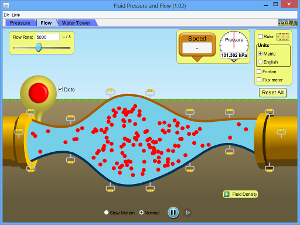Physics Core/AP 1 and 2

#### Course Materials are always under revision! Weblecture content may change anytime prior to two weeks before scheduled chat session for content.Homework

# Physics 10: 7-14 Fluid Mechanics and Bernoulli's Theorem

## Homework

### Reading Preparation

Text Reading: Giancoli, Physics - Principles with Applications, Chapter 10: Sections 8-14

##### Study Points
• 10.8: Because the particles are free to move in different directions, individual particles in flowing liquids and gases do not necessarily follow a nice trajectory, sliding easily past one another in layers. Any interference will cause one or more particles to vere off, creating a chain reaction that results in turbulence. Inherit friction or viscosity between the particles is always present, and becomes greater when turbulence occurs. The amount of fluid that can flow through a cross-sectional area A is determined by the density of the fluid and the rate of speed of the molecules: ρ * A * v. Flow of a incompressible fluid (ρ does not change) under constant pressure through a channel of changing diameter requires the speed to change: A1v1 = A2v2 [The equation of continuity.] As cross-section gets smaller, velocity increases, so that in any identical time interval, the same number of particles pass any point on the channel. If this weren't true, the particles would "back up" like cars in a traffic jam, increasing the density of the liquid -- which isn't allowed in an incompressible liquid.
• 10.9: Now consider an open system, where pressure is allowed to change. If we change the speed of the particles, density changes and pressure changes. Bernoulli's equation shows the relationship of pressure to altitude as air flows across a slanted surface: P1 + ½ρv12 + ρgy1 = P2 + ½ρv22 + ρgy2. We can now compare specific situations against a standard (unmoving air at sea level) or two different situations.
• 10.10: We can now apply Bernoulli's theorem to some common situations. One in particular is the flow of water trhough a small outlet from a large container (such as the flow of water from a reservoir through a dam spillway, or from a storage tank into the main pipe system). In this case, the only pressure is atmospheric pressure, which will be nearly the same at the top and bottom of the contain, so that P1 = P2. If the flow of water at the surface (point 2) is small compared to the flow of water through the spigot (point 1), we can assume v2 ~ 0. Since P1 and P2 cancel, ρ cancels, and v2 can be ignored, Bernoulli's equation simplifies to a form called Torricelli's theorem: ½v12 + gy1 = gy2. We can solve for v1 = sqrt(2g(y2 - y1)).
• 10.11-14: The remaining sections of this chapter discuss applications of these principles to the speed of flow (viscosity), flow within tubes where friction and drag occur, and blood flow in capillaries and through the heart.

### Key Equations

• Equation of continuity:

If the fluid is incompressible (ρ1 = ρ2), rate flow is proportional to the volume of liquid passing through the cross-section A. $A 1 v 1 = A 2 v 2$

• Bernoulli's Principle:
• Poiseuille's equation:

### Web Lecture

Read the following weblecture before chat: Inertia, Acceleration, and Force

### Study Activity

Depending on your browser settings, clicking on the link below will either cause the Java application to download so that you can run it locally, or start te application in the browser.Click to Run

Physics simulation Java Applets are the product of the PHET Interactive Simulations project at the University of Colorado, Boulder.

### Chat Preparation Activities

• Forum question: The Moodle forum for the session will assign a specific study question for you to prepare for chat. You need to read this question and post your answer before chat starts for this session.
• Mastery Exercise: The Moodle Mastery exercise for the chapter will contain sections related to our chat topic. Try to complete these before the chat starts, so that you can ask questions.

### Chapter Quiz

• Required: Complete the Mastery exercise with a passing score of 85% or better.
• Go to the Moodle and take the quiz for this chat session to see how much you already know about astronomy!

### Lab Work

If you want lab credit for this course, you must complete at least 12 labs (honors course) or 18 labs (AP students). One or more lab exercises are posted for each chapter as part of the homework assignment. We will be reviewing lab work at regular intervals, so do not get behind!